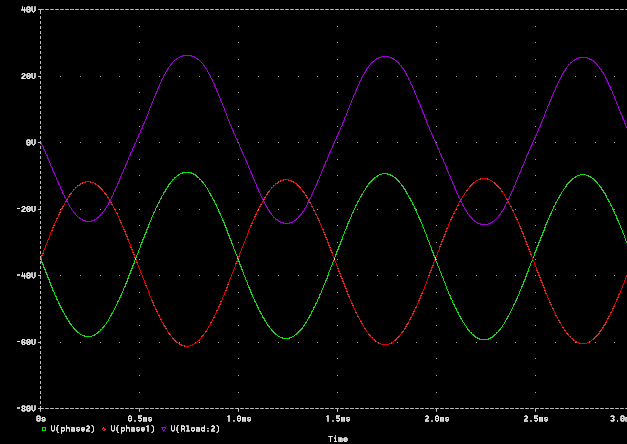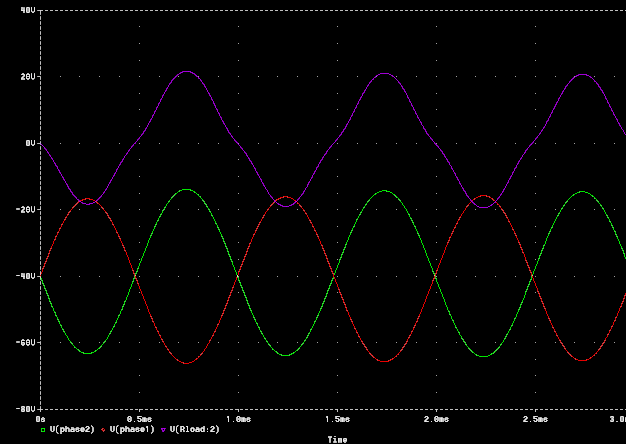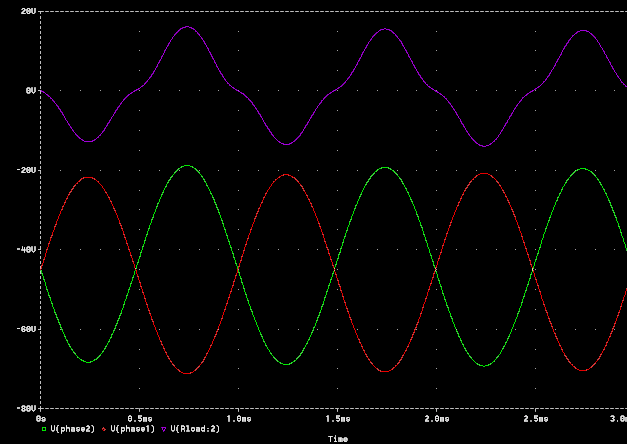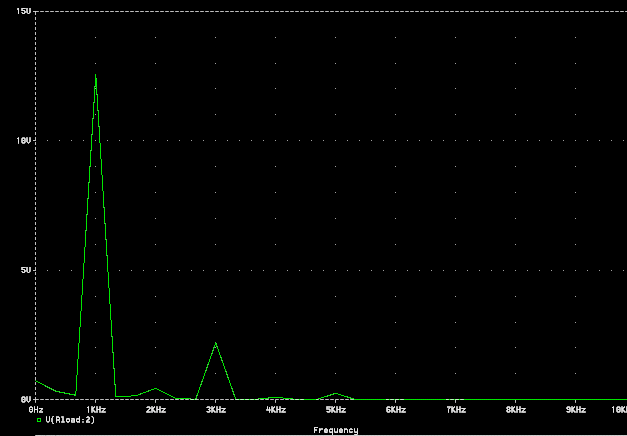What is Crossover Distortion?

General

Crossover distortion is the term given to a type of distortion that occurs in push-pull class AB or class B amplifiers.  It happens during the time that one side of the output stage shuts off, and the other turns on.  Depending upon the bias point, there is a small amount of time where both tubes are in very non-linear portions of their operating curves, or even cut off entirely, and this "kink" in the transfer curves results in a distortion, or notch, at the zero crossing point of the reconstructed waveform.

Examples

The following pictures show how the bias voltage (and thus, the bias current) affects the amount of crossover distortion in a typical 50W push-pull EL34 amplifier.

This first image shows the two drive signals, V(phase1) (the red trace), and V(phase2) ( the green trace), which are 180 degrees out of phase coming from the phase inverter.  The output trace V(Rload:2) (the purple trace), shows the output waveform at the speaker outputs.  As you can see, the negative grid bias voltage is set to -35 volts, as indicated by the average DC level of the two out of phase input signals.  The output waveform shows a fairly decent sine wave with very little crossover distortion at the zero crossing point.This second image shows the effect of increasing the negative bias voltage (which decreases the bias current) to -40V.  You can now see a fair amount of crossover distortion in the output waveform, as indicated by the "kink" in the output trace at the zero crossing of the waveform.This third image shows the effect of increasing the negative bias voltage (which again decreases the bias current) to -45V.  You can now see a large amount of crossover distortion in the output waveform, as indicated by the very large "kink" in the output trace at the zero crossing of the waveform.The next two images show the frequency domain representation of the output waveform, obtained by performing an FFT (fast Fourier transform) on the time domain output data.  This is useful to see the harmonic content of the signal.

As you can see in this first image (corresponding to the FFT of the -35V bias point signal), the output shows a large fundamental component at 1kHz (the frequency of the input sine wave), along with a small blip at 3kHz that is about -30dB down from the fundamental, and another small blip at 5kHz that is about -34dB down, relative to the 25V fundamental signal.  This indicates a small amount of odd order harmonic distortion (even order harmonics would occur at even multiples of the fundamental, such as 2kHz, 4kHz, etc.), which is normal for a push-pull class AB amplifier biased in this region.This second image (corresponding to the FFT of the -45V bias point signal) shows a smaller fundamental, which indicates a lower output level of 12.5V as a result of increasing the negative bias voltage (the bias voltage affects the overall gain).   However,. the 3kHz distortion product is now 2V instead of 0.75V, which is only down -16dB relative to the 12.5V fundamental, and the 5kHz distortion product is now around 0.3V, corresponding to a 5th harmonic distortion level of -32dB.  This indicates that crossover distortion produces mainly third harmonic distortion, which increases rapidly as the negative bias voltage is increased, or as the bias current is decreased.Conclusions

As can be seen from the pictures,  a class AB amplifier can produce a varying amount of crossover distortion, depending upon where the bias point is set.  This crossover distortion is composed of mainly third harmonic distortion, with a bit of fifth harmonic distortion as well.   The amount of distortion that you can "bias out" depends upon the plate voltage the output stage is operating at.  In cases where the plate voltage is very large, you may have to run the bias current very small (corresponding to a larger negative bias voltage) in order to keep the plate dissipation within maximum ratings.  The closer to class B (both tubes biased right at cutoff) you bias the amp, the more power you can get out of it, and the tubes will last longer (unless the amp is played at full power continuously), but the crossover distortion increases drastically.

The lower the plate voltage, the more bias current you can run to reduce the amount of crossover distortion in the output signal. This reduction of plate voltage and increase of plate current converges to the "ideal" point of push-pull class A operation, where the tubes are biased exactly in the middle portion of their transfer characteristics, and neither tube cuts off during any portion of the cycle.  Since the tubes are biased in the most linear portion of their range, there is no pronounced non-linearity at the crossover point, so there is no noticeable crossover distortion.  This is one reason why true class A operation is arguably  "better" than class AB operation.  It is sometimes said that class AB amplifiers are "class A at lower powers", which, while technically correct in terms of conduction angle, is incorrect in terms of the operating point.  The smaller signal swing might not reach the point of plate current cutoff to attain class AB operation, but the tube is still biased in a non-linear portion of the tube characteristics, and some distortion will still be present.

A class AB amplifier usually uses some amount of negative voltage feedback in order to reduce the amount of crossover distortion in the output waveform.  This negative voltage feedback will take out some of the "notch", at least until the point where the output stage is driven to clipping, which opens up the feedback loop because there is no more "excess gain", allowing the full crossover distortion to reappear in the output waveform.

Copyright © 1999,  Randall Aiken.  May not be reproduced in any form without written approval from Aiken Amplification.

Revised 02/18/14